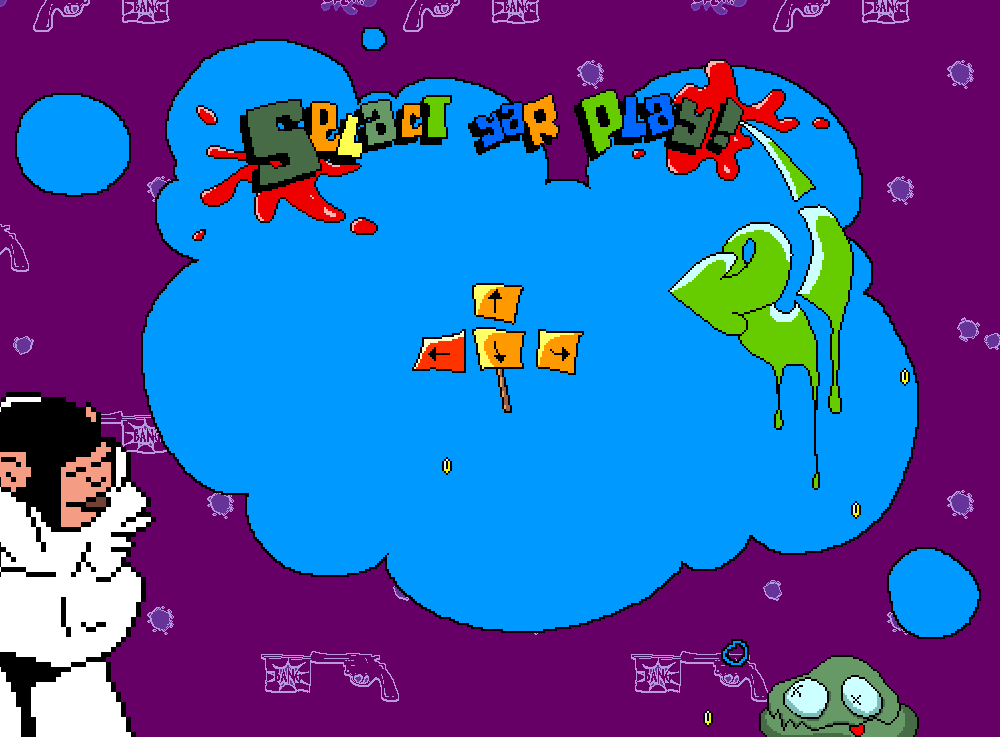# Super Simple Script Snippets: Enemy Projectiles

I’m making a game!
The premise of which is that there will be enemy sprites (like tanks) shooting at you (a spaceship). Enemy projectiles will be fired in short controlled bursts… that’s the basic idea. The real thing is a lot more complex… Imagine short controlled bursts exploding in fireworks that you have to dodge if you don’t manage to “deactivate” them in time with your beam.

Here’s the example (click “Fire!” button):

At any rate I’m releasing code snippets as I progress.
Hopefully these will come in handy for others developing awesome games.

Source code (Actionscript 3 only):

import flash.display.MovieClip;
import flash.events.Event;
import flash.events.MouseEvent;

var num_stageWidth:Number = stage.stageWidth;
var num_stageHeight:Number = stage.stageHeight;

var num_fireAmnt:Number = 10;
txt_amnt.text = num_fireAmnt.toString();

function num_randrange(num_max:Number, num_min:Number = 0)
{
return Math.ceil(Math.random()*(num_max-num_min)+num_min);
}

//vx = x direction (-n is left n is right)
//vy = y direction (how high note: -n is down n is up)
//gravity = gravity on the projectile
//friction = how slow/drug down the projectile is
function makeFire_boss_tank(vx:Number, vy:Number, gravity:Number, friction:Number){
//var mc_boss_clip:MovieClip = MovieClip(stage.getChildByName(“mc_boss”));
var mc_boss_clip:MovieClip = mc_boss;//place holder – comment above in
var mc_bomb:MovieClip = new Fire_Boss_Tank_Bomb();
var arr_data:Array = new Array(vx, vy, gravity, friction);
//put
mc_bomb.data = arr_data;
//placement
mc_bomb.x = mc_boss.x;
mc_bomb.y = mc_boss.y;
//start
}

//trows it up, then down, in a gravity projectile sort of way
function moveFire_boss_tank(event:Event){
var clip:MovieClip = MovieClip(event.currentTarget);
clip.x += (clip.data)/clip.data;
clip.y += (clip.data)/clip.data;
clip.data += clip.data;
//is it off the stage (even if this isn’t likely it’s a good idea…just incase)
if(clip.y>=num_stageHeight){
clip.removeEventListener(Event.ENTER_FRAME,moveFire_boss_tank);
stage.removeChild(clip);
};
}

function initFire_boss_tank(){
//
var num_randFric:Number = num_randrange(1, 3)
var num_randGrav:Number = num_randrange(1, 2);
var num_randvy:Number = num_randrange(-30, -90);
var num_randvx:Number = num_randrange(-15, 15);
//
makeFire_boss_tank(num_randvx, num_randvy, num_randGrav, num_randFric);
}

function fire(event:MouseEvent){
num_fireAmnt = int(txt_amnt.text);